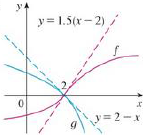Chapter 4.4, Problem 6E### Single Variable Calculus: Early Tr...

8th Edition
James Stewart
ISBN: 9781305270343

#### Solutions

Chapter
Section### Single Variable Calculus: Early Tr...

8th Edition
James Stewart
ISBN: 9781305270343
Textbook Problem

# Use the graphs of f and g and their tangent lines at (2, 0) to find lim x → 2 f ( x ) g ( x ) .To determine

To evaluate: The value of limx2f(x)g(x) by using graphs of f and g.

Explanation

Result used:

L’Hospital’s rule: For differentiable functions f and g where g0 and if the limit limxaf(x)g(x) is in indeterminate form then, limxaf(x)g(x)=limxaf(x)g(x) .

Given:

From the graph it can be identified that the equation of the tangent line of the graph f is y=1.5(x2)

Similarly, the equation of the tangent line of the graph g is y=2x .

Calculation:

Since the equations are the tangents to the curve, it represents its first derivative.

Thus f(x)=1.5(x2) and g(x)=2x

### Still sussing out bartleby?

Check out a sample textbook solution.

See a sample solution

#### The Solution to Your Study Problems

Bartleby provides explanations to thousands of textbook problems written by our experts, many with advanced degrees!

Get Started

#### Find more solutions based on key concepts